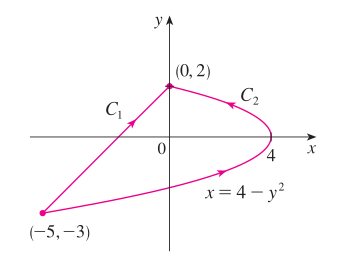Site Overlay

# 矢量微积分 2. 曲线积分、保守场

## 什么是曲线积分？

### 从一元函数积分说起### 一维运动$$\int_{C} f(x, y) d s=\int_{a}^{b} f(x(t), y(t)) \sqrt{\left(\frac{d x}{d t}\right)^{2}+\left(\frac{d y}{d t}\right)^{2}} d t$$

$$\begin{array}\\&\int_{C}\left(2+x^{2} y\right) \mathrm{d} s\\&= \int_0^\pi (2+\cos^2a\sin a) |\langle{\mathrm{d}x},\mathrm{d}y\rangle|\\&= \int_0^\pi (2+\cos^2a\sin a) |{\langle{\mathrm{d}x \over \mathrm{d}t},{\mathrm{d}y \over \mathrm{d}t}\rangle}| \mathrm{d}t\\&= \int_0^\pi (2+\cos^2a\sin a) \sqrt{({\mathrm{d}x \over \mathrm{d}t})^2+({\mathrm{d}y \over \mathrm{d}t})^2} \mathrm{d}t\\&=\int_{0}^{\pi}\left(2+\cos ^{2} t \sin t\right) \sqrt{\sin ^{2} t+\cos ^{2} t} \mathrm{d} t\\&=\int_{0}^{\pi}\left(2+\cos ^{2} t \sin t\right) \mathrm{d} t\\&=\left[2 t-\frac{\cos ^{3} t}\\{3}\right]_{0}^{\pi}\\&=2 \pi+\frac{2}{3}\end{array}$$

### 梯度场曲线积分的计算

【例子】计算 $\int_{C} y^{2} \mathrm{d} x+x \mathrm{d} y$ 。积分路径分别是如图 $C_1, C_2$